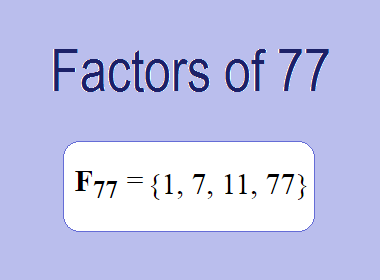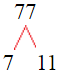# Factors of 77The factors of 77 are 1, 7, 11, and 77 i.e. F77 = {1, 7, 11, 77}. The factors of 77 are all the numbers that can divide 77 without leaving a remainder.

We can check if these numbers are factors of 77 by dividing 77 by each of them. If the result is a whole number, then the number is a factor of 77. Let's do this for each of the numbers listed above:

·        1 is a factor of 77 because 77 divided by 1 is 77.

·        7 is a factor of 77 because 77 divided by 7 is 11.

·        11 is a factor of 77 because 77 divided by 11 is 7.

·        77 is a factor of 77 because 77 divided by 77 is 1.

## How to Find Factors of 77?

1 and the number itself are the factors of every number. So, 1 and 77 are two factors of 77. To find the other factors of 77, we can start by dividing 77 by the numbers between 1 and 77. If we divide 77 by 2, we get a remainder of 1. Therefore, 2 is not a factor of 77. If we divide 77 by 3, we get a remainder of 2. Therefore, 3 is not a factor of 77.

Next, we can check if 4 is a factor of 77. If we divide 77 by 4, we get a remainder of 1. Therefore, 4 is not a factor of 77. We can continue this process for all the possible factors of 77.

Through this process, we can find that the factors of 77 are 1, 7, 11, and 77. These are the only numbers that can divide 77 without leaving a remainder.

********************

********************

## Properties of the Factors of 77

The factors of 77 have some interesting properties. One of the properties is that the sum of the factors of 77 is equal to 96. We can see this by adding all the factors of 77 together:

1 + 7 + 11 + 77 = 96

Another property of the factors of 77 is that they are all odd numbers. This is because 77 is an odd number, and any even number cannot divide an odd number without leaving a remainder.

Another property of the factors of 77 is that the prime factors of 77 are 7 and 11 only.

## Applications of the Factors of 77

The factors of 77 have several applications in mathematics. One of the applications is in finding the highest common factor (HCF) of two or more numbers. The HCF is the largest factor that two or more numbers have in common. For example, to find the HCF of 77 and 28, we need to find the factors of both numbers and identify the largest factor they have in common. The factors of 77 are 1, 7, 11, and 77. The factors of 28 are 1, 2, 4, 7, 14, and 28. The largest factor that they have in common is 7. Therefore, the HCF of 77 and 28 is 7.

Another application of the factors of 77 is in prime factorization. Prime factorization is the process of expressing a number as the product of its prime factors. The prime factors of 77 are 7 and 11 since these are the only prime numbers that can divide 77 without leaving a remainder. Therefore, we can express 77 as:

77 = 7 × 11

We can do prime factorization by division and factor tree method also. Here is the prime factorization of 77 by division method,77 = 7 × 11

Here is the prime factorization of 77 by the factor tree method,77 = 7 × 11

## Conclusion

The factors of 77 are the numbers that can divide 77 without leaving a remainder. The factors of 77 are 1, 7, 11, and 77. The factors of 77 have some interesting properties, such as being odd numbers and having a sum of 96. The factors of 77 have several applications in mathematics, such as finding the highest common factor and prime factorization.